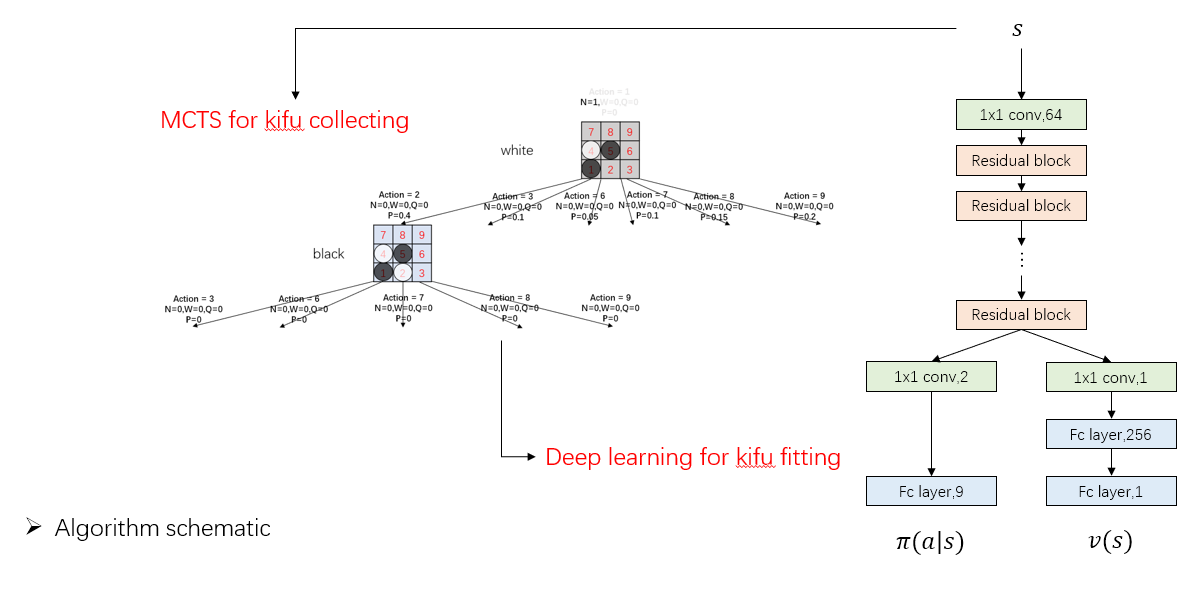# 基于TensorFlow的新型深度学习和强化学习库TensorLayer荐国

Apache

2019-07-31

TensorLayer是一个基于TensorFlow的新型深度学习和强化学习库，专为研究人员和工程师而设计。 它提供了大量可自定义的神经层/功能，这些是构建真实AI应用程序的关键。 TensorLayer被ACM多媒体协会授予2017年最佳开源软件。

• 简单性：TensorLayer将TensorFlow的低级数据流接口提升到高级层/模型。 通过广泛社区提供的丰富示例代码，您可以轻松学习。
• 灵活性：TensorLayer API是透明的：它不会屏蔽用户的TensorFlow; 但留下大量的钩子，有助于低级调整和深度定制。
• 零成本抽象：TensorLayer可以实现TensorFlow的全部功能。 下表显示了使用TensorLayer和TITAN Xp上的原生TensorFlow的VGG16的训练速度。
Mode Lib Data Format Max GPU Memory Usage(MB) Max CPU Memory Usage(MB) Avg CPU Memory Usage(MB) Runtime (sec)
AutoGraph TensorFlow 2.0 channel last 11833 2161 2136 74
Tensorlayer 2.0 channel last 11833 2187 2169 76
Graph Keras channel last 8677 2580 2576 101
Eager TensorFlow 2.0 channel last 8723 2052 2024 97
TensorLayer 2.0 channel last 8723 2010 2007 95

TensorLayer 是作为一个开发库来使用的。 其他包装库如Keras和TFLearn也提供高级抽象。 然而，它们经常隐藏用户的底层引擎，这使得它们难以定制和微调。 相反，TensorLayer API通常是轻量级，灵活且透明的。 用户经常会发现从示例和教程开始很容易，然后无缝地深入了解TensorFlow。 此外，TensorLayer不会通过本机支持创建库锁定，以便从Keras导入组件。

TensorLayer在北京大学，伦敦帝国理工学院，加州大学伯克利分校，卡内基梅隆大学，斯坦福大学，康涅狄格大学（UTC）以及谷歌，微软，阿里巴巴等公司等顶尖研究人员和工程师中的使用增长迅速。

## 模型定义：

``````import tensorflow as tf
from tensorlayer.layers import Input, Dropout, Dense
from tensorlayer.models import Model

def get_model(inputs_shape):
ni = Input(inputs_shape)
nn = Dropout(keep=0.8)(ni)
nn = Dense(n_units=800, act=tf.nn.relu, name="dense1")(nn)
nn = Dropout(keep=0.8)(nn)
nn = Dense(n_units=800, act=tf.nn.relu)(nn)
nn = Dropout(keep=0.8)(nn)
nn = Dense(n_units=10, act=tf.nn.relu)(nn)
M = Model(inputs=ni, outputs=nn, name="mlp")
return M

MLP = get_model([None, 784])
MLP.eval()
outputs = MLP(data)``````

``````class CustomModel(Model):

def __init__(self):
super(CustomModel, self).__init__()

self.dropout1 = Dropout(keep=0.8)
self.dense1 = Dense(n_units=800, act=tf.nn.relu, in_channels=784)
self.dropout2 = Dropout(keep=0.8)#(self.dense1)
self.dense2 = Dense(n_units=800, act=tf.nn.relu, in_channels=800)
self.dropout3 = Dropout(keep=0.8)#(self.dense2)
self.dense3 = Dense(n_units=10, act=tf.nn.relu, in_channels=800)

def forward(self, x, foo=False):
z = self.dropout1(x)
z = self.dense1(z)
z = self.dropout2(z)
z = self.dense2(z)
z = self.dropout3(z)
out = self.dense3(z)
if foo:
out = tf.nn.relu(out)
return out

MLP = CustomModel()
MLP.eval()
outputs = MLP(data, foo=True) # controls the forward here
outputs = MLP(data, foo=False)``````#### 暂无资讯#### 暂无问答

上一篇博客【用tensorflow迁移学习猫狗分类】笔者讲到用tensorlayer的【VGG16模型】迁移学习图像分类，那麽问题来了，tensorlayer没提供的模型怎么办呢？别担心，tensorlayer提供了tenso...

2018/04/29 13:14
18
0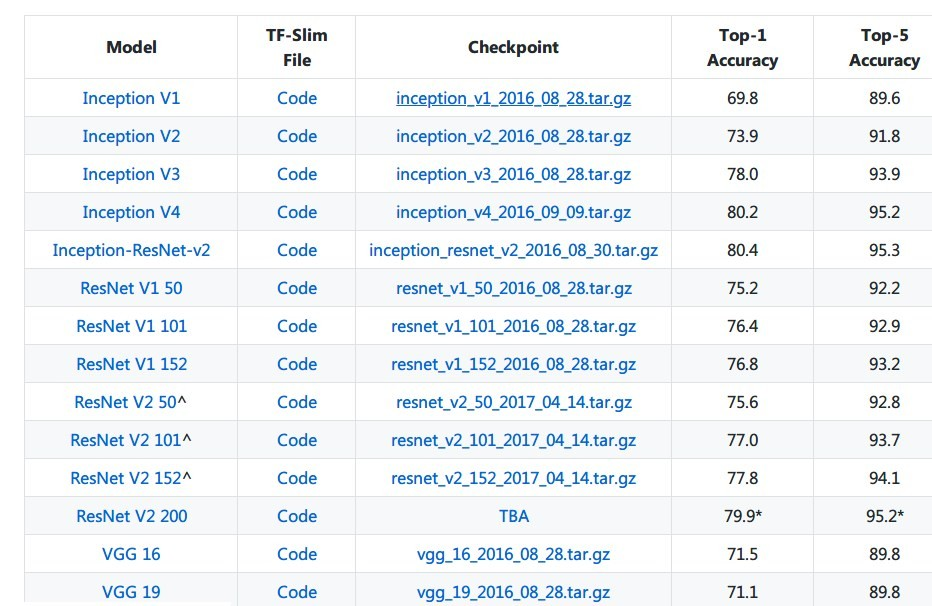(转)如何用TensorLayer做目标检测的数据增强

2018/06/23 09:44
11
0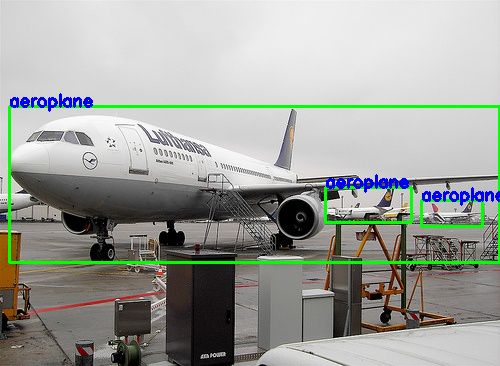2019/04/06 00:41
10
0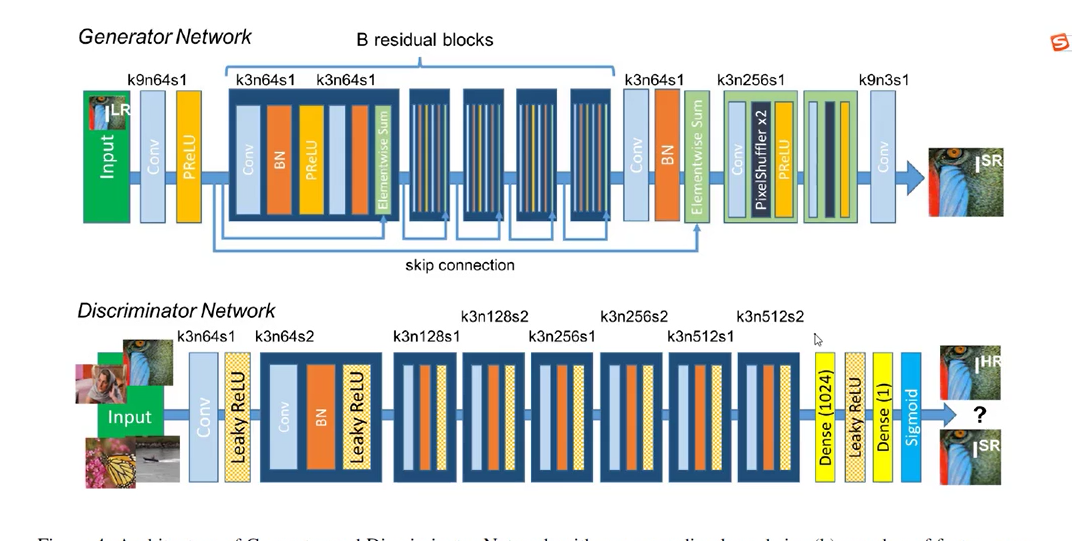2018/06/04 15:09
76
0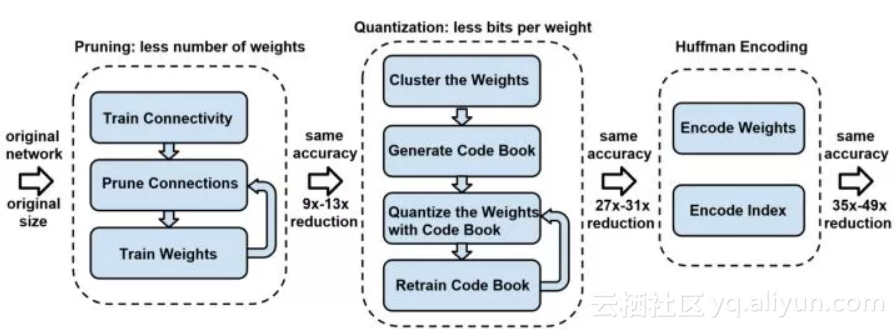TensorFlow的封装

TensorFlow是比较底层的深度学习API，TF-Slim、TFLearn、Keras和TensorLayer均尝试简化TensorFlow/Theano，它们对TensorFlow/Theano的进行高层封装，API实现更加的工程化。从1.0开始，Tenso...

2019/01/18 14:34
15
0

2018/04/19 22:44
43
0

人工智能从 1956 年达特茅斯会议诞生以来，理论和技术发展迅猛。特别是在这波 AI 浪潮中，深度学习的崛起带领 AI 在产业领域的应用范围不断扩大。目前，人工智能已成为新一轮科技革命和产...

07/02 16:23
40
02018/09/12 14:43
32
0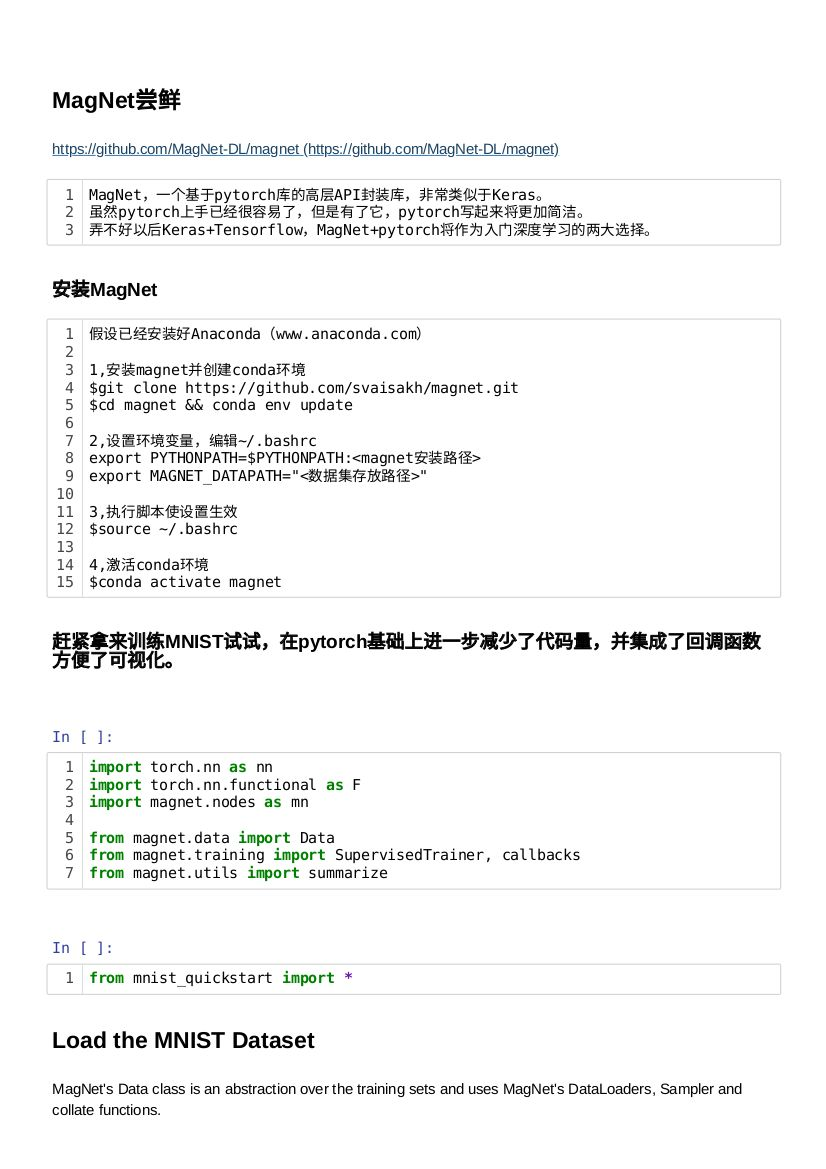AlphaZero并行五子棋AI

AlphaZero-Gomoku-MPI <img src=https://img2018.cnblogs.com/blog/1428973/201812/1428973-20181214131414867-718580985.gif width=400 > Link <font size=5>Github : AlphaZero-Gomoku-MPI...

2018/12/14 13:34
32
0